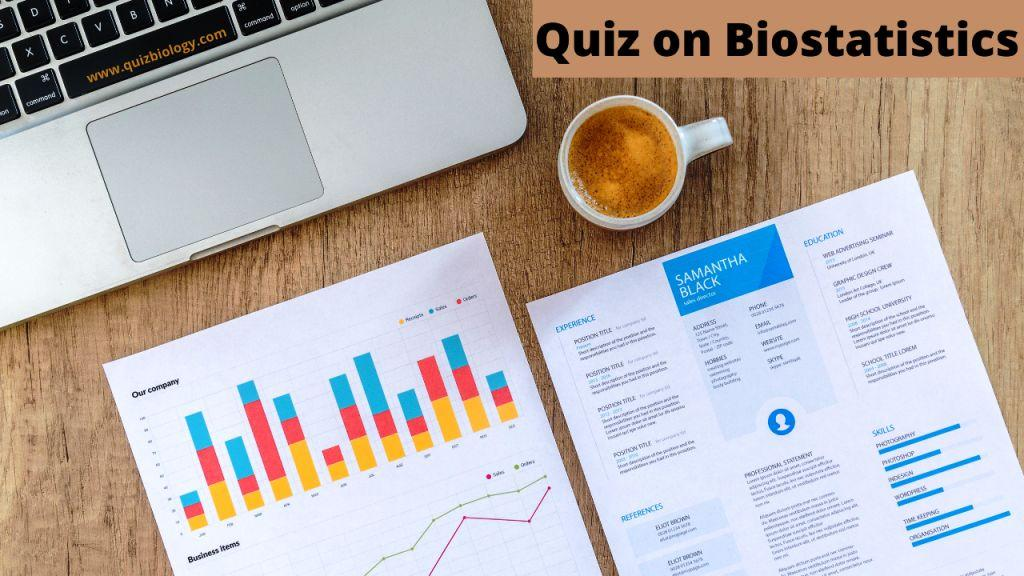# Multiple Choice Quiz on Biostatistics | Biostatistics Quiz1. Who among the following is known as father of biostatistics?
Neyman
Francis Galton
William Gosset

2.Diagram and Graphs are tools of
presentation
collection of data
analysis
None of these

3. Mode can be located graphically with the help oft?
Line diagram
Bar diagram
Histogram
Pie diagram

4. Which of the following is not a measure of central tendency?
Mean
Mode
Median
Range

5. Find the mode in the following data set-11, 12,13,14,14
11
13
12.8
14

6. Standard deviation is the square of
mode
variance
standard error
regression

7. Chi square test
measure the degree of deviation of the experimental result form the expected result
to test the population variance and sample variance
to test the closeness of observed and expected frequency
all of these

8. Chi square is zero when:
expected frequency is lesser than the observed frequency
expected frequency is equal to the observed frequency
expected frequency is double that of the observed frequency
expected frequency is greater than the observed frequency

9. Correlation coefficient is a number between.
-1 and +1
-1 and 0
0 and +1
+1 and +2

10. To determine variation in wing length of butterfly from five different places would be best best statistical test?
F test
Student t test
Regression analysis
Chi square test

Score =